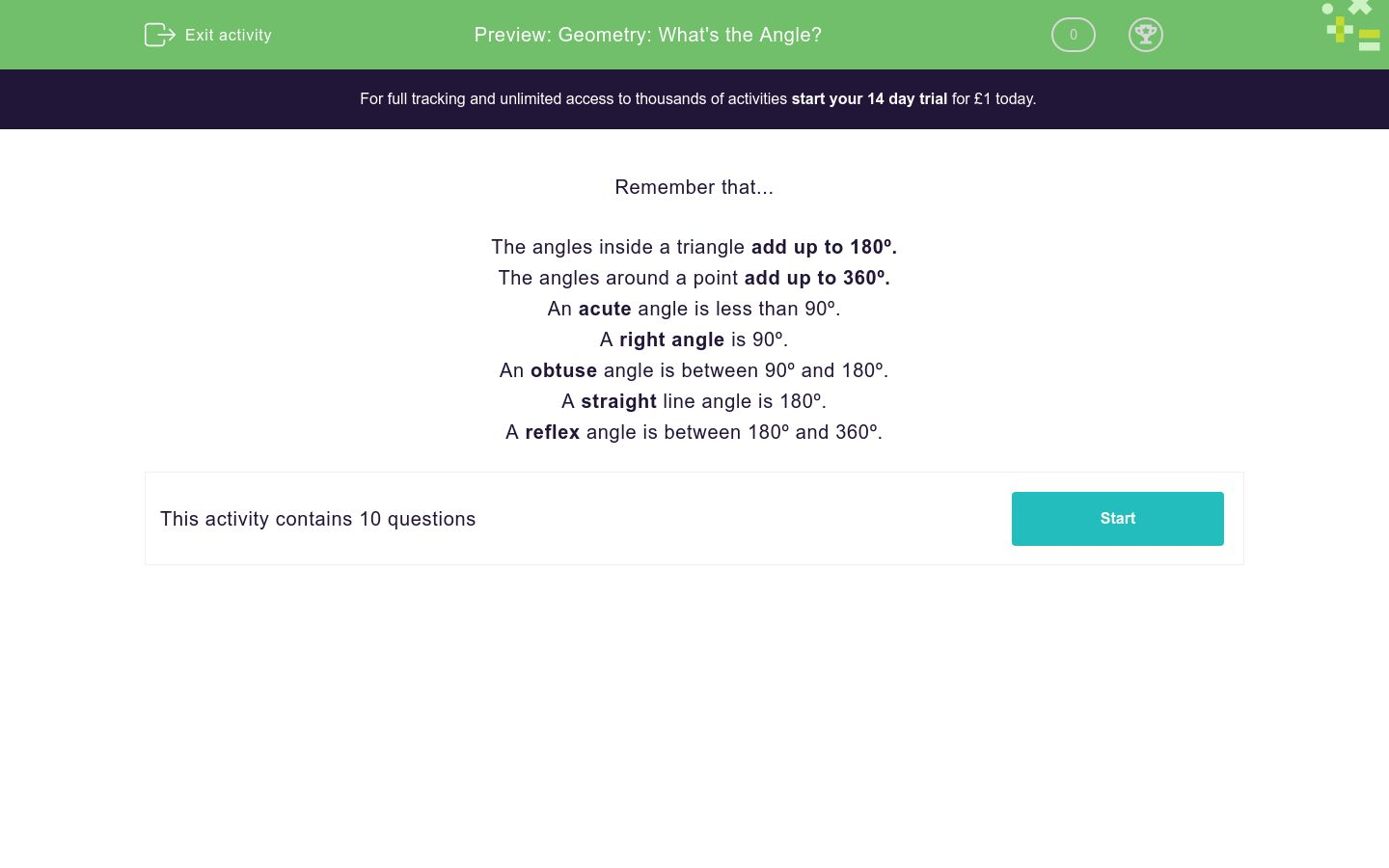# Geometry: What's the Angle?

In this worksheet, students answer a variety of angle-based questions.Key stage:  KS 2

Curriculum topic:   Geometry: Properties of Shapes

Curriculum subtopic:   Compare Shape Properties

Difficulty level:### QUESTION 1 of 10

Remember that...

The angles inside a triangle add up to 180º.

The angles around a point add up to 360º.

An acute angle is less than 90º.

A right angle is 90º.

An obtuse angle is between 90º and 180º.

A straight line angle is 180º.

A reflex angle is between 180º and 360º.

Three of the four angles around a point are 25º , 105º and  65º.

Work out the size of the fourth angle in degrees.

(Just write the number)

Complete the sentence....

A  72º angle is ..................

acute

right angle

obtuse

straight

reflex

Two of the angles in a triangle are 25º and   67º.

Work out the size of the third angle in degrees.

(Just write the number)

Three of the four angles around a point are 255º , 5º and  61º.

Work out the size of the fourth angle in degrees.

(Just write the number)

Complete the sentence....

A  212º angle is ..................

acute

right angle

obtuse

straight

reflex

Two of the angles in a triangle are 115º and   43º.

Work out the size of the third angle in degrees.

(Just write the number)

Two of the three angles around a point are 115º and   43º.

Work out the size of the third angle in degrees.

(Just write the number)

Complete the sentence....

A  142º angle is ..................

acute

right angle

obtuse

straight

reflex

Two of the three angles in a triangle are 79º and   79º.

Work out the size of the third angle in degrees.

(Just write the number)

Four of the five angles around a point are 62º , 63º , 64º and  65º.

Work out the size of the fifth angle in degrees.

(Just write the number)

• Question 1

Three of the four angles around a point are 25º , 105º and  65º.

Work out the size of the fourth angle in degrees.

(Just write the number)

165
EDDIE SAYS
The angles around a point add up to 360º. 25 + 105 + 65 = 195 360 - 195 = 165 The fourth angle is 165.
• Question 2

Complete the sentence....

A  72º angle is ..................

acute
EDDIE SAYS
An angle less than 90º is acute.
• Question 3

Two of the angles in a triangle are 25º and   67º.

Work out the size of the third angle in degrees.

(Just write the number)

88
EDDIE SAYS
The angles inside a triangle add up to 180º. 25 + 67 = 92 180 - 92 = 88 The third angle is 88º.
• Question 4

Three of the four angles around a point are 255º , 5º and  61º.

Work out the size of the fourth angle in degrees.

(Just write the number)

39
EDDIE SAYS
The angles around a point add up to 360º. 255 + 5 + 61 = 321 360 - 321 = 39 The fourth angle is 39.
• Question 5

Complete the sentence....

A  212º angle is ..................

reflex
EDDIE SAYS
A reflex angle is between 180º and 360º.
• Question 6

Two of the angles in a triangle are 115º and   43º.

Work out the size of the third angle in degrees.

(Just write the number)

22
EDDIE SAYS
The angles inside a triangle add up to 180º. 115 + 43 = 158 180 - 158 = 22 The third angle is 22º.
• Question 7

Two of the three angles around a point are 115º and   43º.

Work out the size of the third angle in degrees.

(Just write the number)

202
EDDIE SAYS
The angles around a point add up to 360º. 115 + 43 = 158 360 - 158 = 202 The third angle is 202º.
• Question 8

Complete the sentence....

A  142º angle is ..................

obtuse
EDDIE SAYS
An obtuse angle is between 90º and 180º.
• Question 9

Two of the three angles in a triangle are 79º and   79º.

Work out the size of the third angle in degrees.

(Just write the number)

22
EDDIE SAYS
The angles inside a triangle add up to 180º. 79 + 79 = 158 180 - 158 = 22 The third angle is 22º.
• Question 10

Four of the five angles around a point are 62º , 63º , 64º and  65º.

Work out the size of the fifth angle in degrees.

(Just write the number)

106
EDDIE SAYS
The angles around a point add up to 360º. 62 + 63 + 64 + 65 = 254 360 - 254 = 106 The fifth angle is 106º.
---- OR ----

Sign up for a £1 trial so you can track and measure your child's progress on this activity.

### What is EdPlace?

We're your National Curriculum aligned online education content provider helping each child succeed in English, maths and science from year 1 to GCSE. With an EdPlace account you’ll be able to track and measure progress, helping each child achieve their best. We build confidence and attainment by personalising each child’s learning at a level that suits them.

Get started